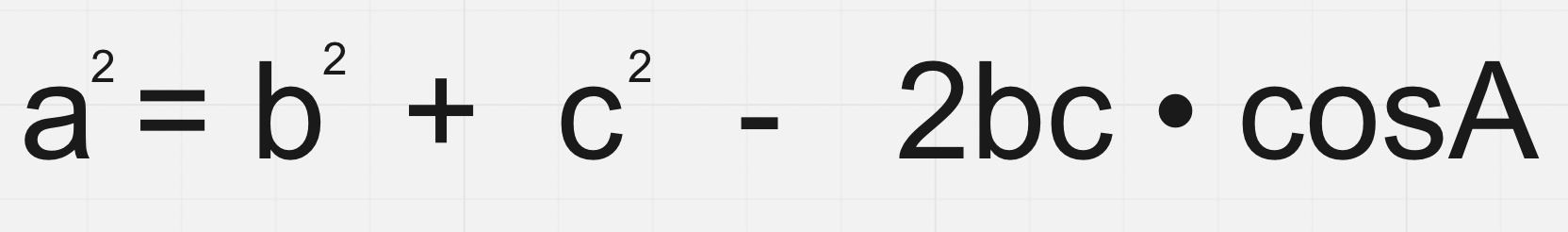Trigonometry: the study of how sides and angles of a triangle are related to each other

# The Right Angle Triangle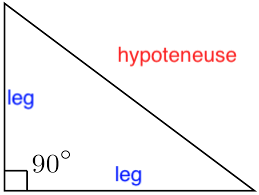• A right angle triangle has a 90º angle

• The side opposing the 90º angle is called the hypotenuse

• the other two sides are called the legs

# Labelling Triangles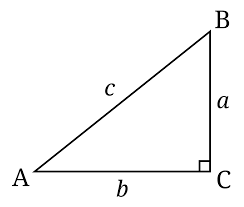• The side opposite an angle and vertex becomes the lowercase letter of that angle and vertex

# Pythagorean theorem

a² + b² = c²

leg² + leg² = hypotenuse²

• used only for side lengths, cannot be used for angles

# Trigonometric Ratios

Primary Trigonometric Ratios

Secondary Trigonometric Ratios

sinθ = O/H

cscθ = H/O

cosθ = A/H

secθ = H/A

tanθ = O/A

cotθ = A/O

• Secondary trig ratios are primary trig ratios, flipped

cscθ = 1/sinθ

secθ = 1/cosθ

cotθ = 1/tanθ

→ you can solve by using the reciprocal when facing a secondary trig ratio

• SOH CAH TOA is the mnemonic device used for primary trig ratios (secondary trig ratios are just flipped)

# Angles of Elevation and Depression

Most often seen in word problems

## Angle of Elevation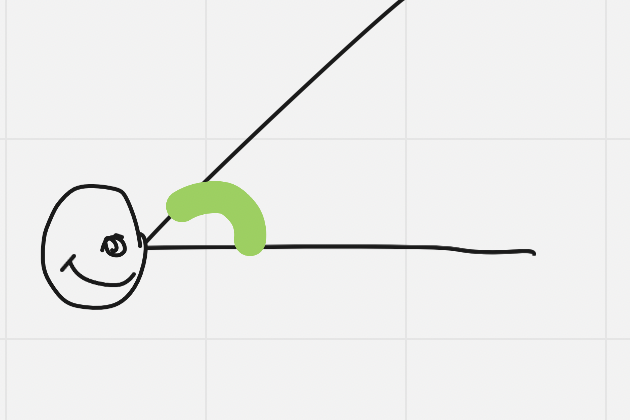The angle between the line of sight and the horizontal from the eye

## Angle of DepressionThe angle when looking down, between the line of sight and the horizontal from the eye

• Because of Z pattern angles, the angle of elevation and depression are actually the same (and are dependent on a question’s phrasing)

# Exact Values

You may not use decimals here, as that is not the true exact value

## Special Triangles

• Used to find the exact values for 45º, 45º isosceles triangles and for 60º, 30º triangles (remember: sum of a triangle’s angles must be 180º)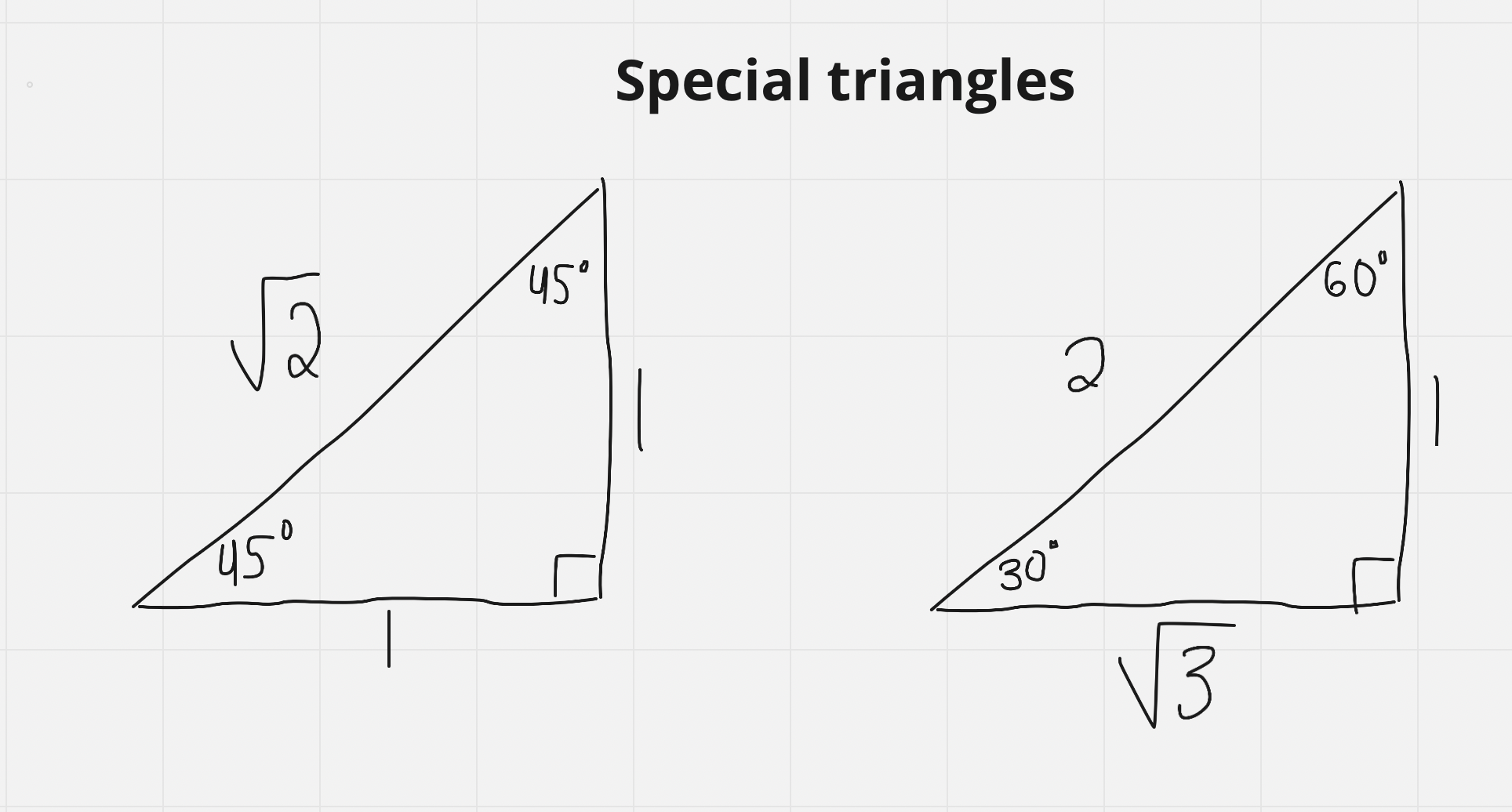# Trig Ratios, Angles Greater Than 90º

• Angles in standard position are always measured from the initial arm to the terminal arm, counterclockwise (arrow always drawn)

• Angles measured closckwise are negative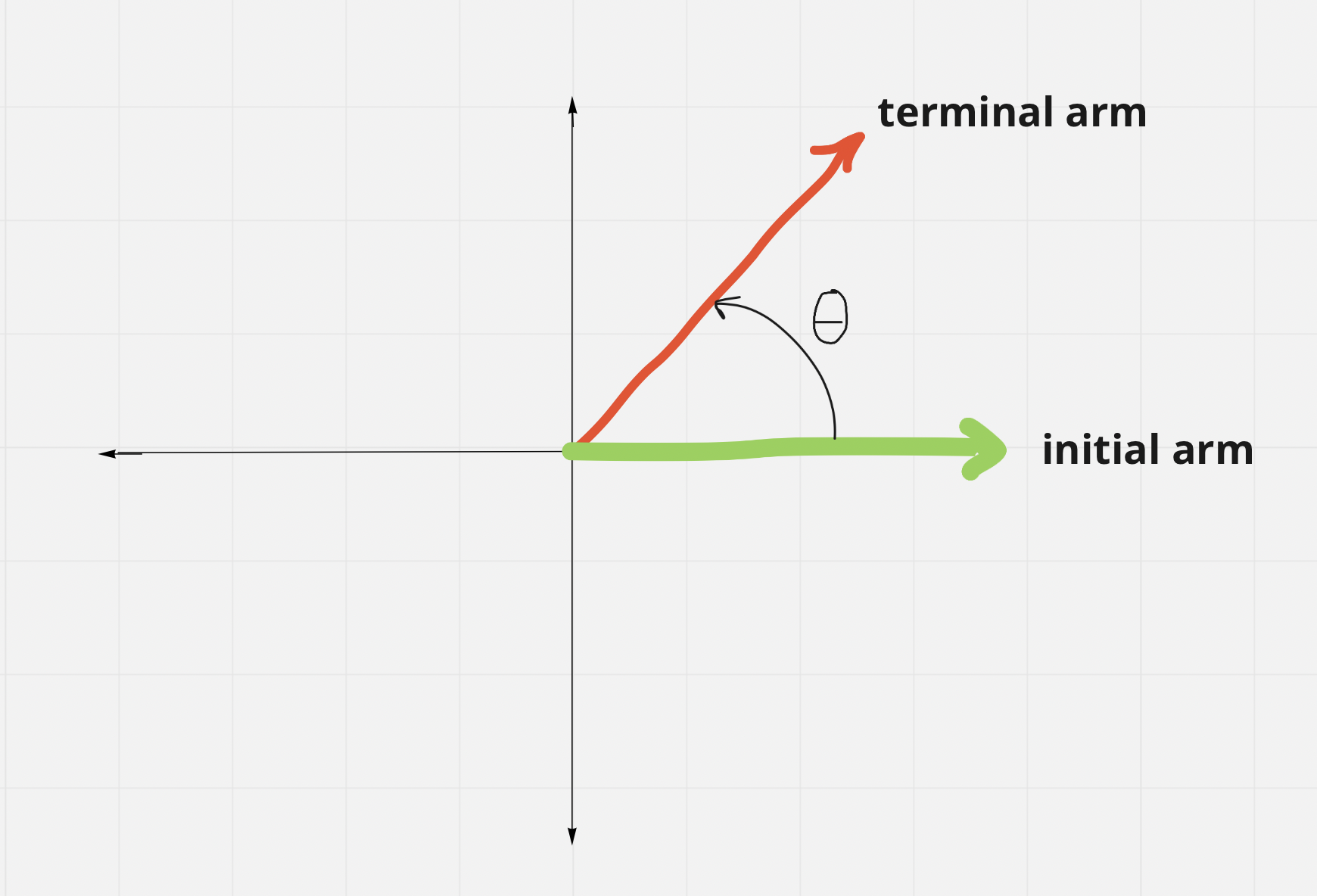The angle, θ, is known as the principal angle (PA)→ its value can fall between 0º and 360º depending on which quadrant the terminal arm is in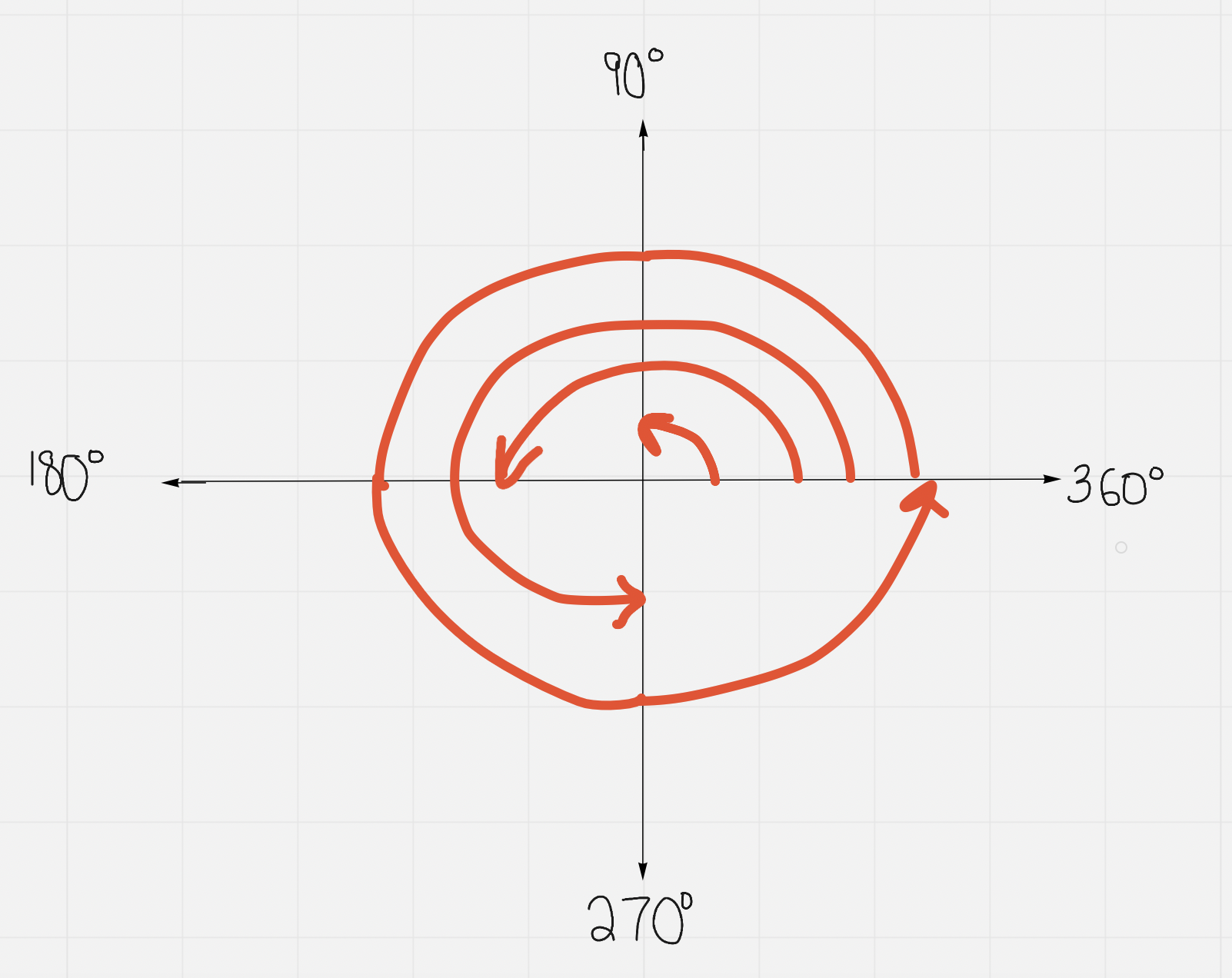The related acute angle (RAA) sits between the terminal arm and the x axis in any quadrant

## RAA and PA in Each Quadrant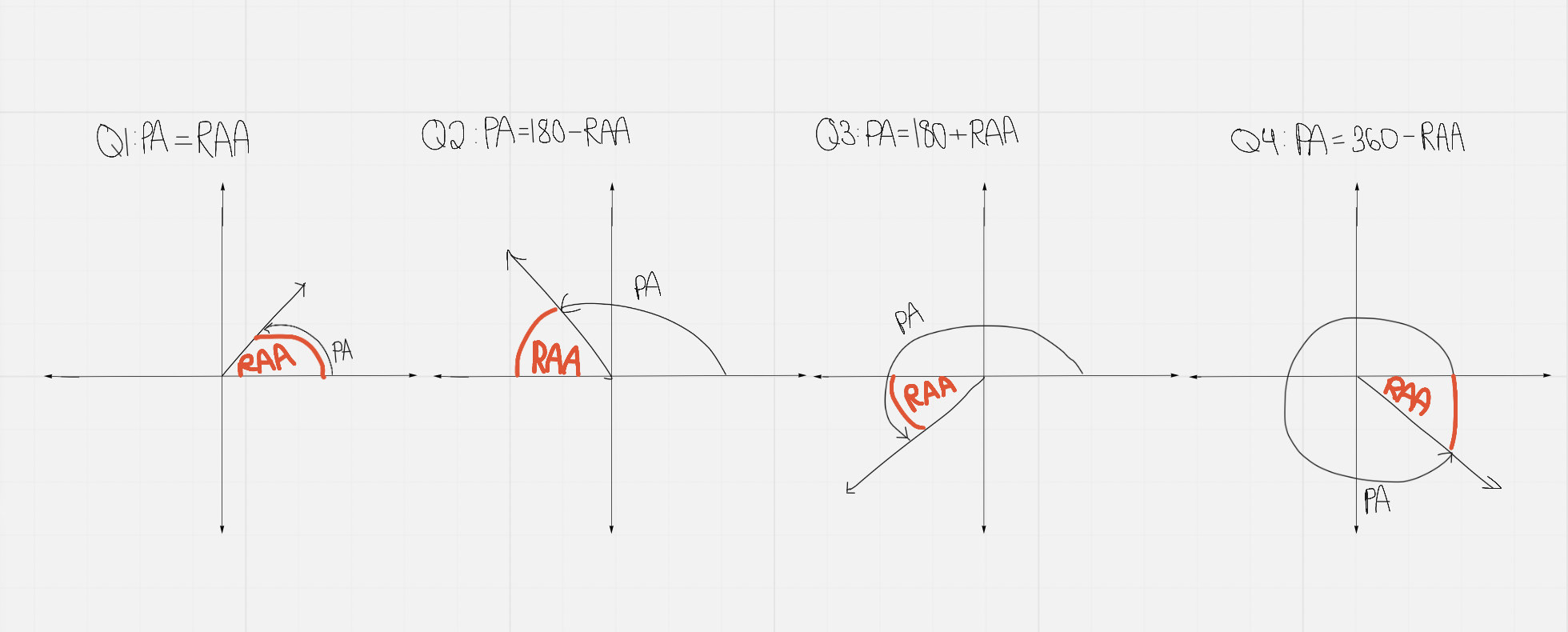## CAST Rule (or: All Students Take Chemistry Rule)

In each quadrant, the given ratio (indicated in the letter) is positive, and in all other quadrants it is negative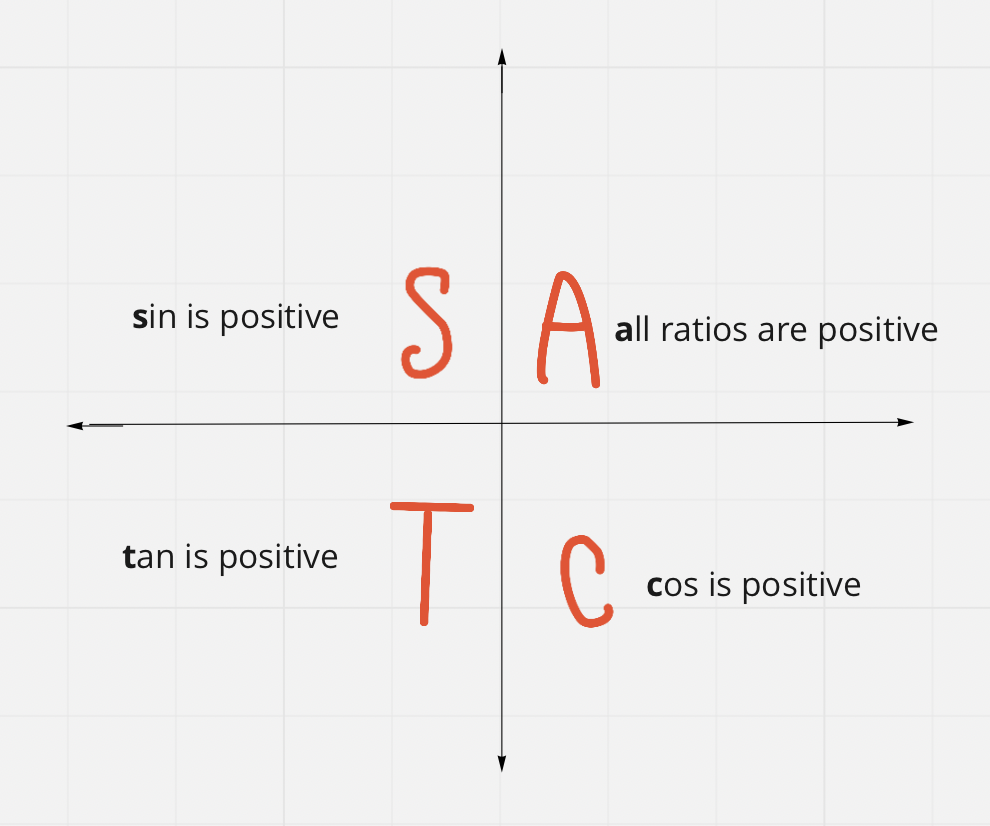## Evaluating Trig Ratios for Any Angle Between 0º and 360º

• You may need to make a triangle (if given a point that the terminal line intersects through, for instance) and so you may need to use this tool to solve the problem

• If a point (ordered pair) is given, then x and y are accounted for as you know what they are from looking at the point which is formatted (x,y)

Apply the formula used to find the circumference of a circle to SOH CAH TOA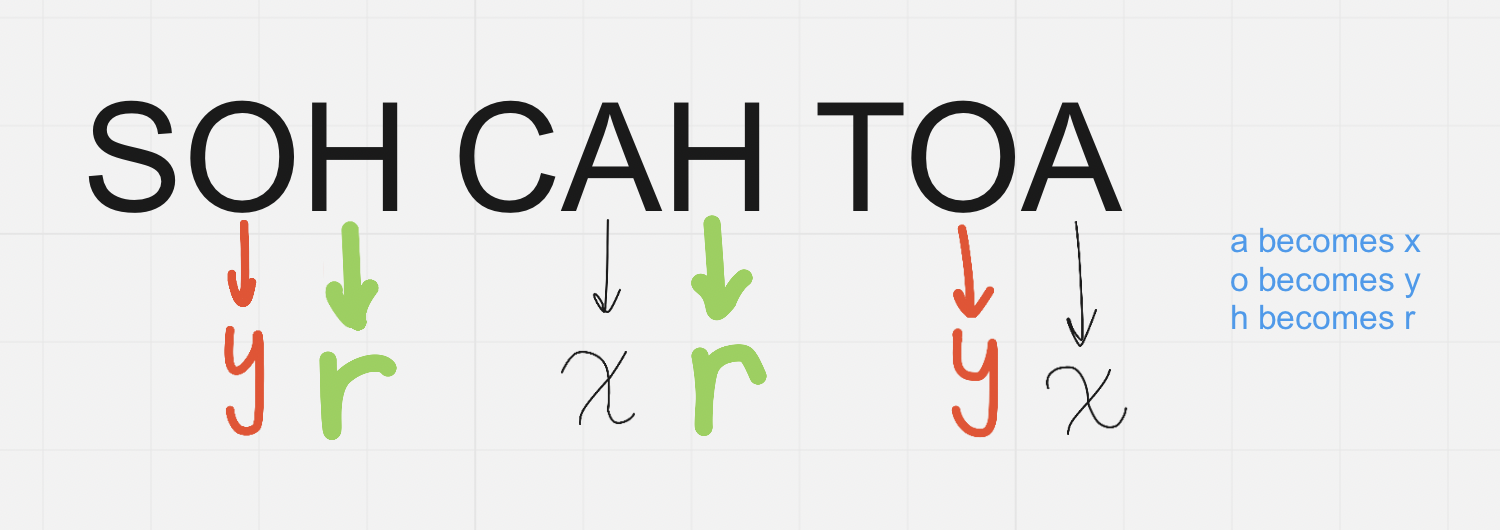1. Use the Pythagorean theorem (adapted to circle, same formula → x² + y² = r² ) to get the missing value

2. Use a primary trig ratio to find the RAA:

ratio⁻¹(side O, A, H depending on question/side O, A, H depending on question) = RAA

3. Use RAA to calculate PA (dependent on the quad, Recall: RAA and PA in Each Quadrant graphic above)

# Non-right Angle Triangles

For triangle ABC:

## Sine Law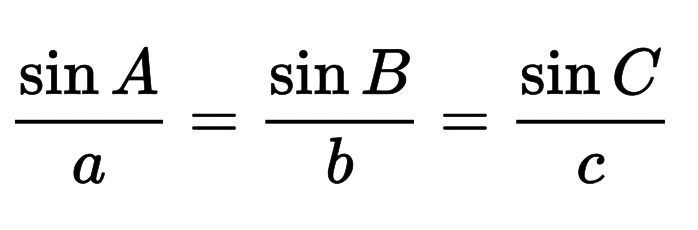## Cosine Law